### Introduction

Self-Organizing Maps was developed as a neural network technique for dimensionality reduction and to act as a clustering algorithm. It is also known as Kohonen Map, named after the famous professor Teuvo Kohonen and is abbreviated as SOM. Kohonen maps follow a competitive algorithm for unsupervised learning applications. A self-organizing map has only two layers in the neural network wherein the first layer (input layer) will have the number of neurons equal to the number of features in the dataset, and the second layer (output layer) will have the number of neurons equal to the number of clusters that the user want.

### Working of SOM

Self-organizing maps take a dataset as its input and form clusters based on the weight matrix assigned to it. The input layer forms a complete network mesh with the output layer. This system of neural network layers can be considered to be a weight matrix. For ‘n’ features and ‘k’ clusters, there are n rows and k columns for the weight matrix.Each column in a weight matrix is called a vector, which corresponds to a cluster. When a sample is passed through the weight matrix, the distance between each sample element with respect to the weights of each vector.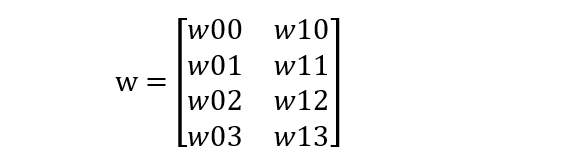The weight matrix is assigned with random values initially. The winning vector for a particular sample is that vector that has the minimum distance from the sample. This vector is also called the Best Matching Unit for the sample input.After each sample is trained, the winning vector is updated using the learning rate of the algorithm (α) which is usually set to 0.5 in conventional cases. Any test sample gets clustered by finding out the distance of the vector to the sample.This aspect of SOMs makes it different from the other neural networks which require backpropagation with gradient descent, making it a competitive algorithm.

### Algorithm

1. Decide the number of clusters based on the size of the dataset and the number of features.

2. Create the weight matrix.

3. For each training sample, compute the distance from each vector and find the winning vector.

4. Update the weights of the winning vector after each sample is trained.

5. Iterate the steps mentioned above for the number of epochs specified.

6. Use the final weight matrix for clustering the test sample.

### Implementation

Let us consider an example with the following unlabelled samples and segregate them into clusters.1. Based on the size of the data and the number of features present (4 features), we can create 2 clusters. Therefore, our weight matrix will be 4×2 in dimension. We will iterate it for only 1 epoch.

2. We can create a weight matrix with random weights as follows: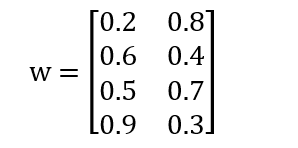3. Using the aforementioned formulae to find the distance of the first sample with the two vectors and finding the winning vector: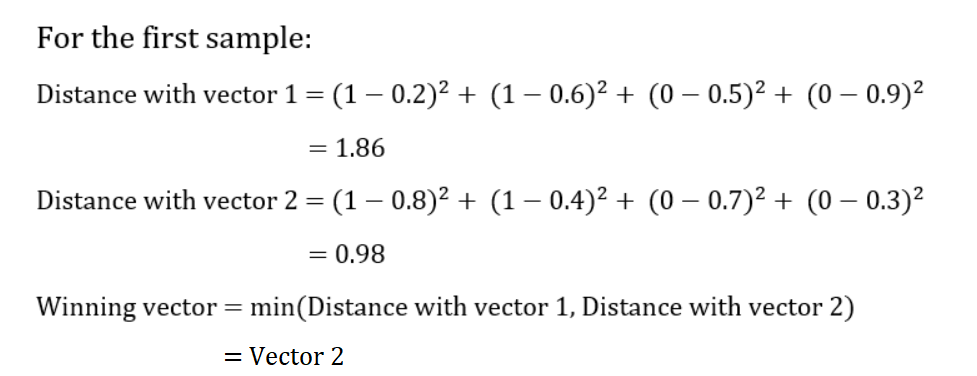4. Since vector 2 is the winning vector, we update its weights: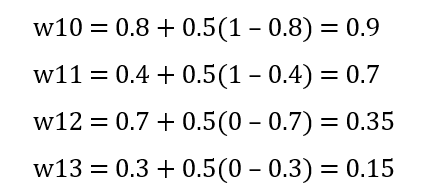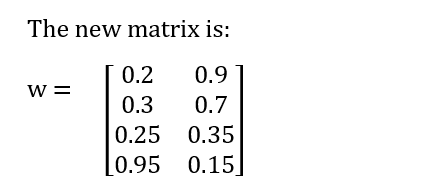This is continued for all the other training samples. The next training sample always trains with the new weight matrix and updates it, as given below: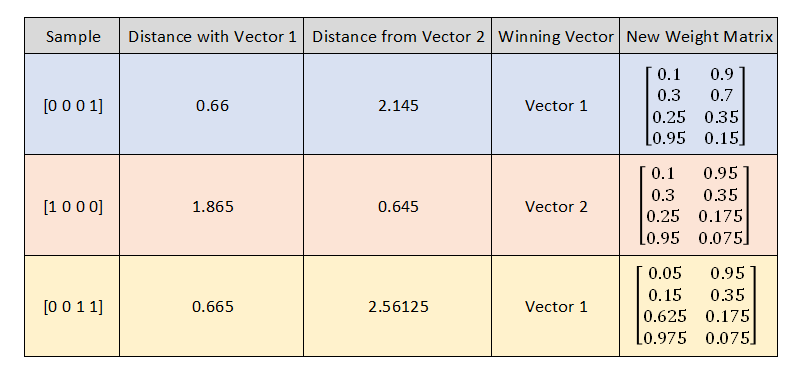The final weight matrix is: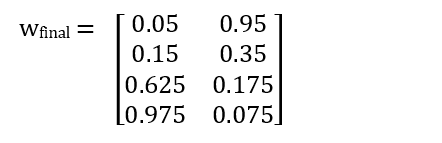6. Let us consider a test sample [1 0 1 0] that needs to be clustered. Finding the distances:Let us code the algorithm of SOM by creating a function that takes the samples as inputs and returns the final weights as the output. The weights can be then used to pass through a prediction function to put the samples into their respective clusters.

				
fun selforganizingmap(arr: Array,n: Int,cluster: Int): Array
{
val w = Array(cluster) { DoubleArray(n) }
val min = 0.01
val max = 0.99
val alpha = 0.5
for (i in 0 until cluster)
{
for (j in 0 until n)
{
w[i][j] = Math.random() * (max - min) + min
}
}
println("Initial weights: ")
for (i in w.indices)
{
for (j in w.indices)
{
print(w[i][j].toString() + " ")
}
println()
}
val v = DoubleArray(cluster)
for (i in arr.indices)
{
for (j in 0 until cluster)
{
for (k in 0 until n)
{
v[j] += Math.pow(arr[i][k] - w[j][k], 2.0)
}
}
var min_index = 0
for (k in 0 until cluster)
{
if (v[k] &lt; v[min_index])
{
min_index = k
}
}
for (k in 0 until n)
{
w[min_index][k] = w[min_index][k] + alpha * (arr[i][k] - w[min_index][k])
}
}
return w
}


				
val arr = arrayOf(
doubleArrayOf(1.0, 1.0, 0.0, 0.0),
doubleArrayOf(0.0, 0.0, 0.0, 1.0),
doubleArrayOf(1.0, 0.0, 0.0, 0.0),
doubleArrayOf(0.0, 0.0, 1.0, 1.0)
)
val ans = selforganizingmap(arr, arr.size, 2)
println("Final weights: ")
for (i in ans.indices)
{
for (j in ans.indices)
{
print(ans[i][j].toString() + " ")
}
println()
}



Output:

				
Initial weights:
0.39400559765840093 0.7722927705594362 0.32036243515111795 0.741893482240412
0.45534821840966244 0.33793991112761845 0.4100230685672652 0.9048796977889698
Final weights:
0.6970027988292005 0.8861463852797181 0.16018121757555898 0.370946741120206
0.30691852730120783 0.04224248889095231 0.5512528835709081 0.7381099622236212



Note: Since the weights are being randomly generated, the cluster formation might change every time the code is executed, and therefore the cluster sample combinations might differ. Also, the epoch in this function is set to 1. However, a simple loop can iterate this procedure for the number of epochs given.

				
fun predictvector(test: DoubleArray, weights: Array): Int
{
val v = DoubleArray(weights.size)
for (i in weights.indices)
{
for (j in test.indices)
{
v[i] += Math.pow(test[j] - weights[i][j], 2.0)
}
}
var min_index = 0
for (i in weights.indices)
{
if (v[i] &lt; v[min_index])
{
min_index = i
}
}
return min_index
}


				
val test = doubleArrayOf(1.0, 0.0, 1.0, 0.0)
val classify = predictvector(test, ans)
println("Test data lies under cluster: " + (classify + 1))



Output:

				
Test data lies under cluster: 2